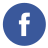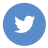### java date and time

java

Java provides the java.time package for working with dates and times. This package replaces the old java.util.Date and java.util.Calendar classes.

The java.time package includes the following main classes:

• `LocalDate` represents a date (year, month, day) without a time.
• `LocalTime` represents a time (hour, minute, second, nanosecond) without a date.
• `LocalDateTime` represents a date and time without a time zone.
• `ZonedDateTime` represents a date and time with a time zone.
• `Duration` represents a duration (e.g., “2 hours and 30 minutes”)
• `Period` represents a period (e.g., “2 years and 3 months”)

Here’s an example of how you can use these classes to perform some common tasks:

``````import java.time.LocalDate;
import java.time.LocalTime;
import java.time.LocalDateTime;
import java.time.ZonedDateTime;
import java.time.Duration;
import java.time.Period;

public class Main {
public static void main(String[] args) {
// Get the current date and time
LocalDateTime current = LocalDateTime.now();
System.out.println("Current date and time: " + current);

// Get the current date
LocalDate date = current.toLocalDate();
System.out.println("Current date: " + date);

// Get the current time
LocalTime time = current.toLocalTime();
System.out.println("Current time: " + time);

// Get the current date and time in a specific time zone
ZonedDateTime zoned = ZonedDateTime.now();
System.out.println("Current date and time in time zone: " + zoned);

// Get the difference between two dates and times
LocalDateTime past = LocalDateTime.of(2000, 1, 1, 0, 0, 0);
Duration duration = Duration.between(past, current);
System.out.println("Duration: " + duration);

// Get the difference between two dates
LocalDate pastDate = LocalDate.of(2000, 1, 1);
Period period = Period.between(pastDate, date);
System.out.println("Period: " + period);
}
}``````